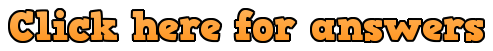Workout

Question 1:

(a)  1 < x < 4

(b)  -1 < x < 2

(c)  -7 ≤ x  ≤ -3

(d)  -4 ≤ x  ≤ 5

(e)  x < 0  or  x > 9

(f)  x < -6  or  x > 5

(g)  x ≤ -10  or  x ≥ -1

(h)  x ≤ -7  or  x ≥ 7

(i)    -8 < x < -2

(j)    x ≤ -7  or  x ≥ 4

(k)   -1 ≤ x  ≤ 5

(l)    x < 12  or  x > 11

Question 2

(a)  x < -3  or  x > -2

(b)  -5 < x < -2

(c)   -1 ≤ x  ≤ 5

(d)  x <-6   or  x >4

(e)    x ≤ 2  or  x ≥ 4

(f)    -4 < x < 1

(g)  x < -7  or  x > 8

(h)   – 6 < x < -3

(i)      2 ≤ x  ≤ 11

(j)    – 4 < x < 8

(k)   x ≤ -8  or  x ≥ 8

(l)   x < 6  or  x > 8

Question 3:

(a)   -3 < x < 5

(b)   x < -4   or  x > -1

(c)   -6 < x < 6

(d)  x < -11  or  x > 11

(e)    -4 ≤ x  ≤ 3

(f)      4 < x < 10

(g)     -18 < x < 2

(h)     x ≤ -4  or  x ≥ 6

Question 4:

(a)     x < 3   or  x > 5

(b)      -2 ≤ x  ≤ 5

(c)       x ≤ -5  or  x ≥ -1

(d)       2 < x < 16

(e)       x < -4  or  x > 11

(f)        x < -1   or  x > 0

Question 5:

(a)     0.5 < x < 4

(b)     x < -4   or  x > -0.2

(c)      -3.5 ≤ x  ≤ 8

(d)       -1 ≤ x  ≤ 0.66666…

(e)        x < 0.75   or  x > 4.5

(f)        x ≤ -0.6666…  or  x ≥ 1.666…

Question 6:

(a)        x < -1   or    x > -0.4

(b)        – 3 < x < 0.3333…

(c)        x < 0.5   or    x > 4

(d)        x ≤ -0.25  or  x ≥ 1

(e)        1 < x < 1.1666666..

(f)         -2 ≤ x  ≤ 1.5

(g)      x < 0.75   or  x > 2

(h)     x ≤ 0.75  or  x ≥ 6

(i)     -1.666… < x < 1.4

Apply

Question 1:

Mistake 1 – Tia really should sketch a graph (or use a table/number line depending on your own preference)

Mistake 2 – The answer should be  x < -3   or  x > 11.  A graph would help Tia with that  🙂

Question 2:

(a)   x = -3 + √6    or   x = -3 – √6

(b)  -3 – √6 < x < -3 + √6

Question 3:    x² + 7x + 10 < 0

Question 4:    x² – 3x – 18 > 0

Question 5:     1.5 < x < 10

Question 6:     (2x + 1)(2x – 3) > 0

4x² – 4x – 3 > 0

Question 7:     50 < x < 110